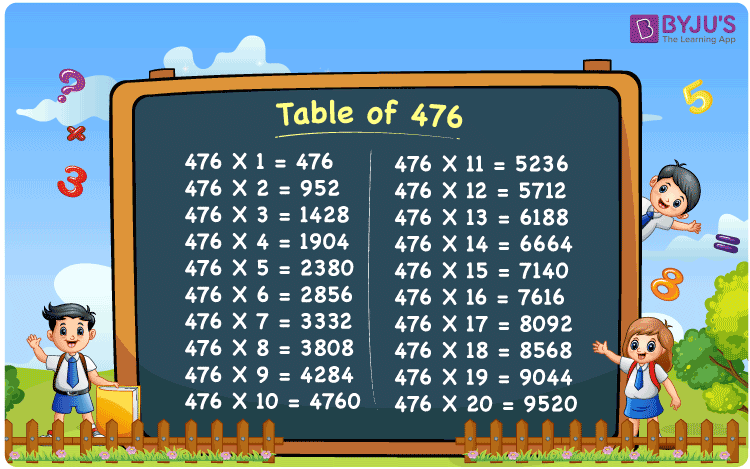Checkout JEE MAINS 2022 Question Paper Analysis : Checkout JEE MAINS 2022 Question Paper Analysis :

# Table of 476

The table of 476 is presented here for the convenience of students. It is extremely important to learn them because direct questions based on multiples of 476 are often asked in competitive exams. The multiplication table of 476 is obtained by multiplying 476 with natural numbers. This article offers the table of 476 in tabular form as well as a PDF file for offline access.

## Table of 476 Chart## What is the 476 Times Table?

The table of 476, sometimes known as the 476 times table, depicts the addition of the number 476 for a certain number of times.

For example, 476 × 5 = 2380, which is the same as 476 + 476 + 476 + 476 + 476 = 2380.

 476×1 = 476 476 476×2 = 952 476 + 476 = 952 476×3 = 1428 476 + 476 + 476 = 1428 476×4 = 1904 476 + 476 + 476 + 476 = 1904 476×5 = 2380 476 + 476 + 476 + 476 + 476 = 2380 476×6 = 2856 476 + 476 + 476 + 476 + 476 + 476 = 2856 476×7 = 3332 476 + 476 + 476 + 476 + 476 + 476 + 476 = 3332 476×8 = 3808 476 + 476 + 476 + 476 + 476 + 476 + 476 + 476 = 3808 476×9 = 4284 476 + 476 + 476 + 476 + 476 + 476 + 476 + 476 + 476 = 4284 476×10 = 4760 476 + 476 + 476 + 476 + 476 + 476 + 476 + 476 + 476 + 476 = 4760

## Multiplication Table of 476

The table below shows the multiplication table of 476 in tabular form:

 476 × 1 = 476 476 × 2 = 952 476 × 3 = 1428 476 × 4 = 1904 476 × 5 = 2380 476 × 6 = 2856 476 × 7 = 3332 476 × 8 = 3808 476 × 9 = 4284 476 × 10 = 4760 476 × 11 = 5236 476 × 12 = 5712 476 × 13 = 6188 476 × 14 = 6664 476 × 15 = 7140 476 × 16 = 7616 476 × 17 = 8092 476 × 18 = 8568 476 × 19 = 9044 476 × 20 = 9520

## Solved Example on the Table of 476

Example:

What is 60 more than 20 times 476?

Solution:

Using the table of 476, 20 times 476 is 9520.

I.e., 20 × 476 = 9520.

Hence, 60 more than 20 times 476 = 60 + 9520 = 9580.

## Frequently Asked Questions on the Table of 476

### What is the table of 476?

476×1 = 476, 476×2 = 952, 476×3 = 1428, 476×4 = 1904, 476×5 = 2380, 476×6 = 2856, 476×7 = 3332, 476×8 = 3808, 476×9 = 4284, 476×10 = 4760.

### How many times does 476 equal 3332?

7 times 476 equals 3332.

### What is 476 times 14?

476 times 14 is 6664. I.e., 476 × 14 = 6664.